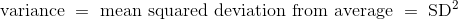# 十三、预测

``````# Galton's data on heights of parents and their adult children
heights = Table().with_columns(
'MidParent', galton.column('midparentHeight'),
'Child', galton.column('childHeight')
)
heights
``````
MidParent Child
75.43 73.2
75.43 69.2
75.43 69
75.43 69
73.66 73.5
73.66 72.5
73.66 65.5
73.66 65.5
72.06 71
72.06 68

（省略了 924 行）

``````heights.scatter('MidParent')
``````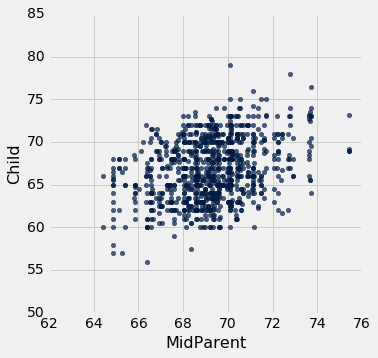``````def predict_child(mpht):
"""Return a prediction of the height of a child
whose parents have a midparent height of mpht.

The prediction is the average height of the children
whose midparent height is in the range mpht plus or minus 0.5 inches.
"""

close_points = heights.where('MidParent', are.between(mpht-0.5, mpht + 0.5))
return close_points.column('Child').mean()
``````

``````# Apply predict_child to all the midparent heights

heights_with_predictions = heights.with_column(
'Prediction', heights.apply(predict_child, 'MidParent')
)
# Draw the original scatter plot along with the predicted values

heights_with_predictions.scatter('MidParent')
``````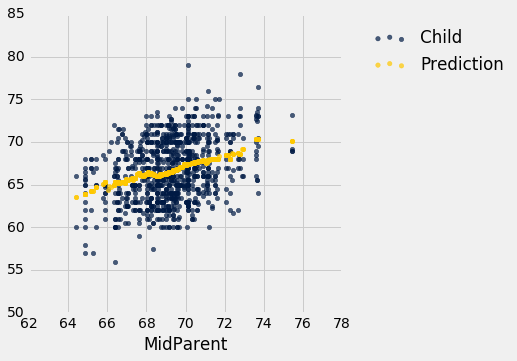## 相关性

`hybrid`表包含了 1997 年到 2013 年在美国销售的混合动力车的数据。数据来自佛罗里达大学 Larry Winner 教授的在线数据档案。这些列为：

• `vehicle`：车的型号
• `year`：出厂年份
• `msrp`: 2013 年制造商的建议零售价（美元）
• `acceleration`: 加速度（千米每小时每秒）
• `mpg`: 燃油效率（英里每加仑）
• `class`: 型号的类别

（省略了 143 行）

``````hybrid.scatter('acceleration', 'msrp')
``````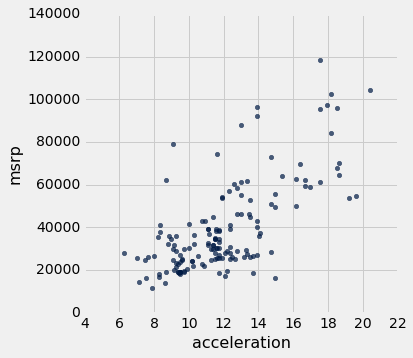`msrp``mpg`的散点图表明了负相关。 `mpg`较高的混合动力车往往成本较低。 这似乎令人惊讶，直到你明白了，加速更快的汽车往往燃油效率更低，行驶里程更低。 之前的散点图显示，这些也是价格更高的车型。

``````hybrid.scatter('mpg', 'msrp')
````````````suv = hybrid.where('class', 'SUV')
suv.scatter('mpg', 'msrp')
``````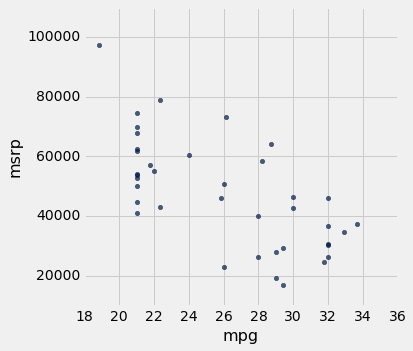``````suv.scatter('acceleration', 'msrp')
````````````def standard_units(any_numbers):
"Convert any array of numbers to standard units."
return (any_numbers - np.mean(any_numbers))/np.std(any_numbers)
``````

``````Table().with_columns(
'mpg (standard units)',  standard_units(suv.column('mpg')),
'msrp (standard units)', standard_units(suv.column('msrp'))
).scatter(0, 1)
plots.xlim(-3, 3)
plots.ylim(-3, 3);
``````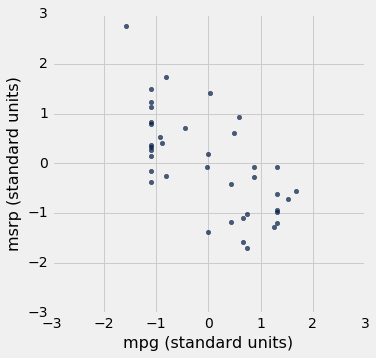``````Table().with_columns(
'acceleration (standard units)', standard_units(suv.column('acceleration')),
'msrp (standard units)',         standard_units(suv.column('msrp'))
).scatter(0, 1)
plots.xlim(-3, 3)
plots.ylim(-3, 3);
``````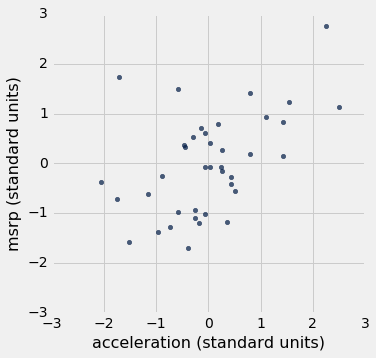### 相关系数

• 相关系数`r`是介于`-1``1`之间的数字。
• `r`度量了散点图围绕一条直线聚集的程度。
• 如果散点图是完美的向上倾斜的直线，`r = 1`，如果散点图是完美的向下倾斜的直线，`r = -1`

`r = 1`时，散点图是完全线性的，向上倾斜。 当`r = -1`时，散点图是完全线性的，向下倾斜。 当`r = 0`时，散点图是围绕水平轴的不定形云，并且变量据说是不相关的。

``````r_scatter(0.9)
``````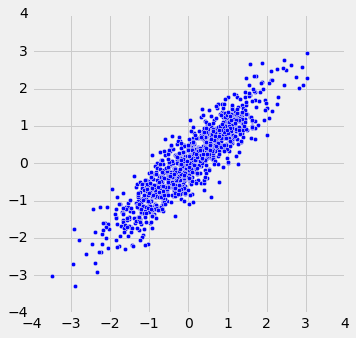``````r_scatter(0.25)
``````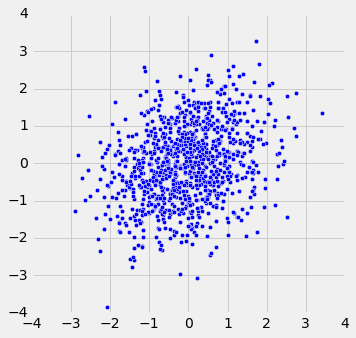``````r_scatter(0)
````````````r_scatter(-0.55)
``````### 计算`r`

`r`的公式：

`r`是两个变量的乘积的均值，这两个变量都以标准单位来衡量。

``````x = np.arange(1, 7, 1)
y = make_array(2, 3, 1, 5, 2, 7)
t = Table().with_columns(
'x', x,
'y', y
)
t
``````
x y
1 2
2 3
3 1
4 5
5 2
6 7

``````t.scatter(0, 1, s=30, color='red')
````````````t_su = t.with_columns(
'x (standard units)', standard_units(x),
'y (standard units)', standard_units(y)
)
t_su
``````
x y x (standard units) y (standard units)
1 2 -1.46385 -0.648886
2 3 -0.87831 -0.162221
3 1 -0.29277 -1.13555
4 5 0.29277 0.811107
5 2 0.87831 -0.648886
6 7 1.46385 1.78444

``````t_product = t_su.with_column('product of standard units', t_su.column(2) * t_su.column(3))
t_product
``````
x y x (standard units) y (standard units) product of standard units
1 2 -1.46385 -0.648886 0.949871
2 3 -0.87831 -0.162221 0.142481
3 1 -0.29277 -1.13555 0.332455
4 5 0.29277 0.811107 0.237468
5 2 0.87831 -0.648886 -0.569923
6 7 1.46385 1.78444 2.61215

``````# r is the average of the products of standard units

r = np.mean(t_product.column(4))
r
0.61741639718977093
``````

### `r`的性质

`r`是一个纯数字。 它没有单位。 这是因为`r`基于标准单位。 `r`不受任何轴上单位的影响。 这也是因为`r`基于标准单位。 `r`不受轴的交换的影响。 在代数上，这是因为标准单位的乘积不依赖于哪个变量被称为`x``y`。 在几何上，轴的切换关于`y = x`直线翻转了散点图，但不会改变群聚度和关联的符号。

``````t.scatter('y', 'x', s=30, color='red')
``````### `correlation`函数

``````def correlation(t, x, y):
return np.mean(standard_units(t.column(x))*standard_units(t.column(y)))
``````

``````correlation(t, 'x', 'y')
0.61741639718977093
``````

``````correlation(t, 'y', 'x')
0.61741639718977093
``````

`suv`表的列上调用`correlation`，可以使我们看到价格和效率之间的相关性，以及价格和加速度之间的相关性。

``````correlation(suv, 'mpg', 'msrp')
-0.6667143635709919
correlation(suv, 'acceleration', 'msrp')
0.48699799279959155
``````

### 相关性度量线性关联

``````new_x = np.arange(-4, 4.1, 0.5)
nonlinear = Table().with_columns(
'x', new_x,
'y', new_x**2
)
nonlinear.scatter('x', 'y', s=30, color='r')
``````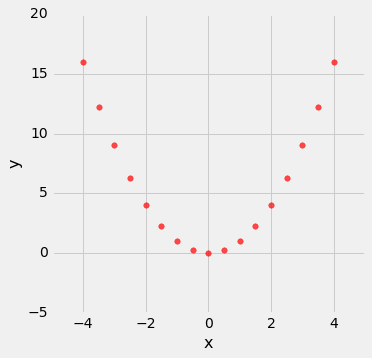``````correlation(nonlinear, 'x', 'y')
0.0
``````

### 相关性受到离群点影响

``````line = Table().with_columns(
'x', make_array(1, 2, 3, 4),
'y', make_array(1, 2, 3, 4)
)
line.scatter('x', 'y', s=30, color='r')
````````````correlation(line, 'x', 'y')
1.0
outlier = Table().with_columns(
'x', make_array(1, 2, 3, 4, 5),
'y', make_array(1, 2, 3, 4, 0)
)
outlier.scatter('x', 'y', s=30, color='r')
``````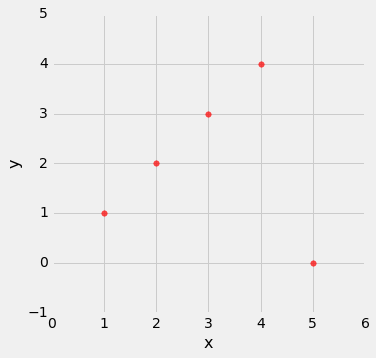``````correlation(outlier, 'x', 'y')
0.0
``````

### 生态相关性应谨慎解读

``````sat2014 = Table.read_table('sat2014.csv').sort('State')
sat2014
``````
State Participation Rate Critical Reading Math Writing Combined
Alabama 6.7 547 538 532 1617
Alaska 54.2 507 503 475 1485
Arizona 36.4 522 525 500 1547
Arkansas 4.2 573 571 554 1698
California 60.3 498 510 496 1504
Colorado 14.3 582 586 567 1735
Connecticut 88.4 507 510 508 1525
Delaware 100 456 459 444 1359
District of Columbia 100 440 438 431 1309
Florida 72.2 491 485 472 1448

（省略了 41 行）

``````sat2014.scatter('Critical Reading', 'Math')
``````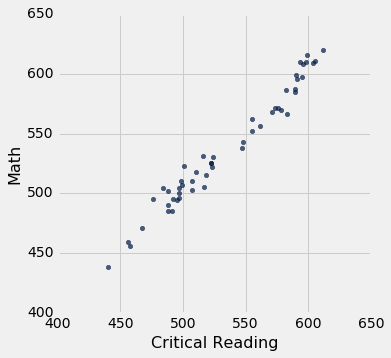``````correlation(sat2014, 'Critical Reading', 'Math')
0.98475584110674341
``````

### 严重还是开玩笑？

2012 年，在著名的《新英格兰医学杂志》（New England Journal of Medicine）上发表的一篇论文，研究了一组国家巧克力消费与的诺贝尔奖之间的关系。《科学美国人》（Scientific American）严肃地做出回应，而其他人更加轻松。 欢迎你自行决定！下面的图表应该让你有兴趣去看看。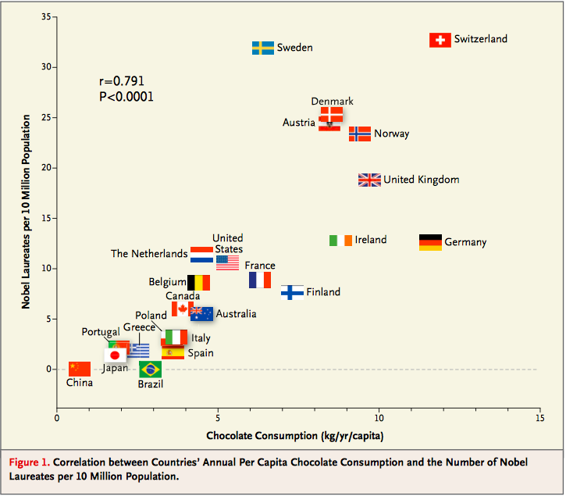## 回归直线

``````galton = Table.read_table('galton.csv')

heights = Table().with_columns(
'MidParent', galton.column('midparentHeight'),
'Child', galton.column('childHeight')
)
def predict_child(mpht):
"""Return a prediction of the height of a child
whose parents have a midparent height of mpht.

The prediction is the average height of the children
whose midparent height is in the range mpht plus or minus 0.5 inches.
"""

close_points = heights.where('MidParent', are.between(mpht-0.5, mpht + 0.5))
return close_points.column('Child').mean()
heights_with_predictions = heights.with_column(
'Prediction', heights.apply(predict_child, 'MidParent')
)
heights_with_predictions.scatter('MidParent')
``````### 标准单位下的度量

``````def standard_units(xyz):
"Convert any array of numbers to standard units."
return (xyz - np.mean(xyz))/np.std(xyz)
heights_SU = Table().with_columns(
'MidParent SU', standard_units(heights.column('MidParent')),
'Child SU', standard_units(heights.column('Child'))
)
heights_SU
``````
MidParent SU Child SU
3.45465 1.80416
3.45465 0.686005
3.45465 0.630097
3.45465 0.630097
2.47209 1.88802
2.47209 1.60848
2.47209 -0.348285
2.47209 -0.348285
1.58389 1.18917
1.58389 0.350559

（省略了 924 行）

``````sd_midparent = np.std(heights.column(0))
sd_midparent
1.8014050969207571
0.5/sd_midparent
0.27756111096536701
``````

``````def predict_child_su(mpht_su):
"""Return a prediction of the height (in standard units) of a child
whose parents have a midparent height of mpht_su in standard units.
"""
close = 0.5/sd_midparent
close_points = heights_SU.where('MidParent SU', are.between(mpht_su-close, mpht_su + close))
return close_points.column('Child SU').mean()
heights_with_su_predictions = heights_SU.with_column(
'Prediction SU', heights_SU.apply(predict_child_su, 'MidParent SU')
)
heights_with_su_predictions.scatter('MidParent SU')
``````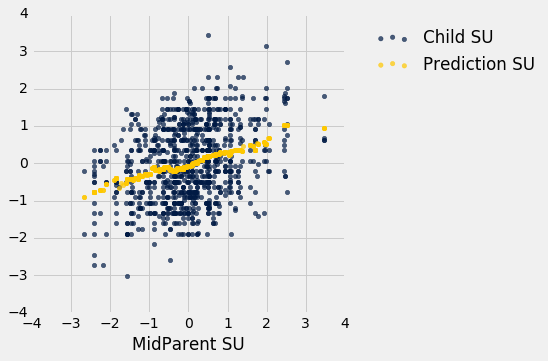### 确定标准单位下的直线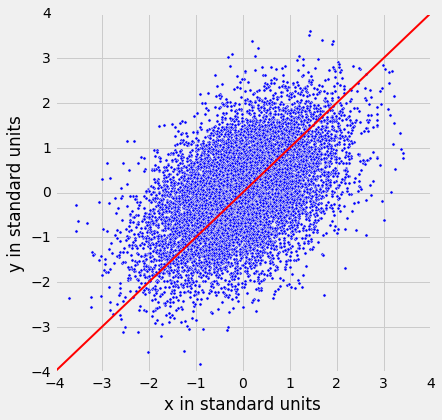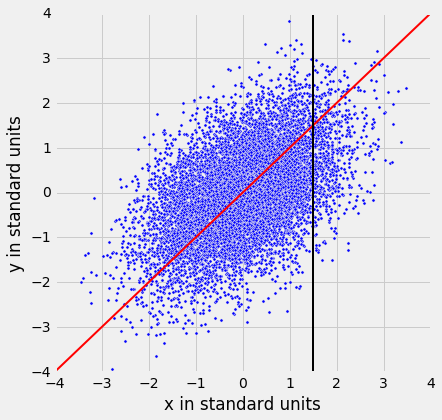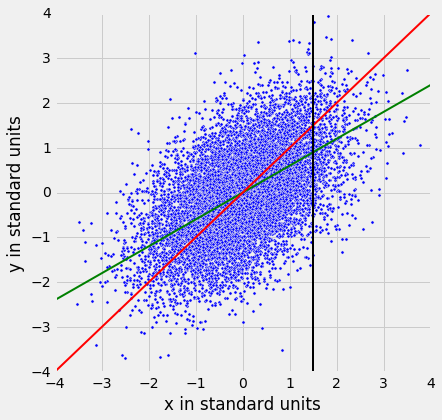45 度线的斜率为 1。所以绿色的“均值图”直线的斜率是正值但小于 1。

### 标准单位下的回归直线

``````regression_line(0.95)
``````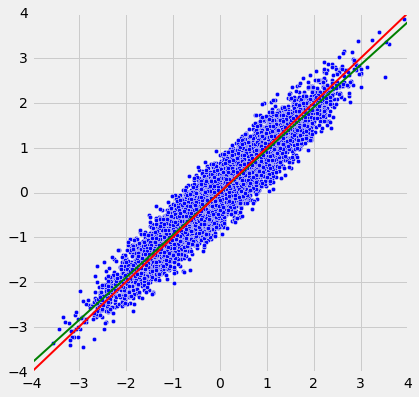``````regression_line(0.6)
``````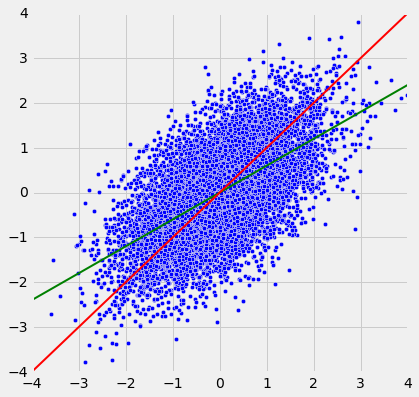`r`接近于 1 时，散点图，45 度线和回归线都非常接近。 但是对于`r`较低值来说，回归线显然更平坦。

### 回归直线的方程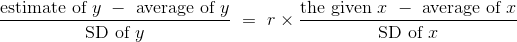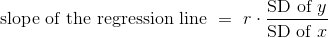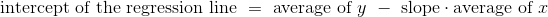``````def correlation(t, label_x, label_y):
return np.mean(standard_units(t.column(label_x))*standard_units(t.column(label_y)))

def slope(t, label_x, label_y):
r = correlation(t, label_x, label_y)
return r*np.std(t.column(label_y))/np.std(t.column(label_x))

def intercept(t, label_x, label_y):
return np.mean(t.column(label_y)) - slope(t, label_x, label_y)*np.mean(t.column(label_x))
``````

### 回归直线和高尔顿的数据

``````galton_r = correlation(heights, 'MidParent', 'Child')
galton_r
0.32094989606395924
``````

``````galton_slope = slope(heights, 'MidParent', 'Child')
galton_intercept = intercept(heights, 'MidParent', 'Child')
galton_slope, galton_intercept
(0.63736089696947895, 22.636240549589751)
``````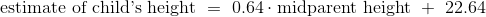``````galton_slope*70.48 + galton_intercept
67.557436567998622
``````

``````heights_with_predictions.where('MidParent', are.equal_to(70.48)).show(3)
``````
MidParent Child Prediction
70.48 74 67.6342
70.48 70 67.6342
70.48 68 67.6342

（省略了 5 行）

``````heights_with_predictions = heights_with_predictions.with_column(
'Regression Prediction', galton_slope*heights.column('MidParent') + galton_intercept
)
heights_with_predictions
``````
MidParent Child Prediction Regression Prediction
75.43 73.2 70.1 70.7124
75.43 69.2 70.1 70.7124
75.43 69 70.1 70.7124
75.43 69 70.1 70.7124
73.66 73.5 70.4158 69.5842
73.66 72.5 70.4158 69.5842
73.66 65.5 70.4158 69.5842
73.66 65.5 70.4158 69.5842
72.06 71 68.5025 68.5645
72.06 68 68.5025 68.5645

（省略了 924 行）

``````heights_with_predictions.scatter('MidParent')
``````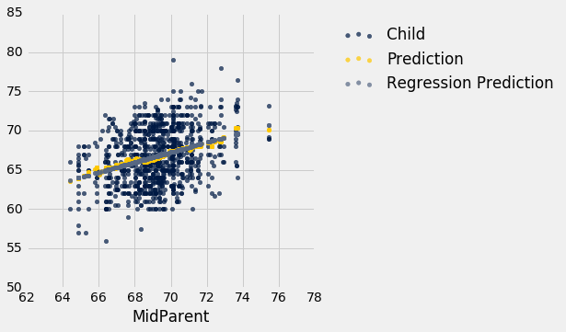### 拟合值

``````def fit(table, x, y):
"""Return the height of the regression line at each x value."""
a = slope(table, x, y)
b = intercept(table, x, y)
return a * table.column(x) + b
``````

``````heights.with_column('Fitted', fit(heights, 'MidParent', 'Child')).scatter('MidParent')
``````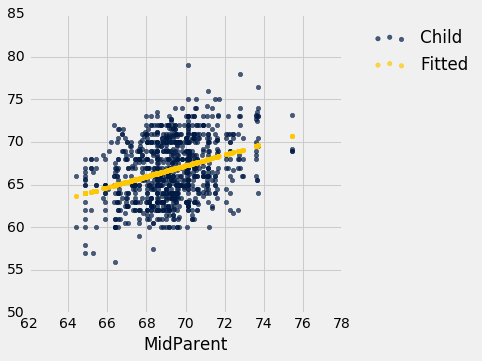``````heights.scatter('MidParent', fit_line=True)
``````### 斜率的测量单位

``````baby = Table.read_table('baby.csv')
baby.scatter('Maternal Height', 'Maternal Pregnancy Weight', fit_line=True)
``````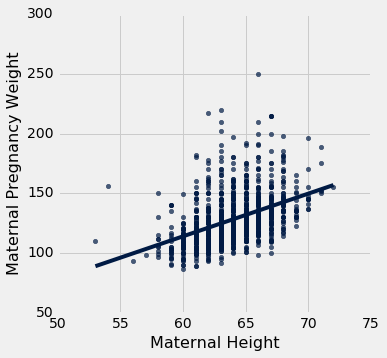``````slope(baby, 'Maternal Height', 'Maternal Pregnancy Weight')
3.5728462592750558
``````

### 示例

average SD
height 14 inches 2 inches
weight 50 pounds 5 pounds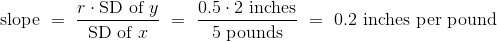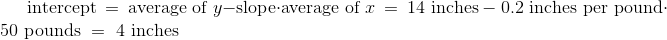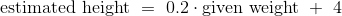## 最小二乘法

``````little_women = Table.read_table('little_women.csv')
little_women = little_women.move_to_start('Periods')
little_women.show(3)
``````
Periods Characters
189 21759
188 22148
231 20558

（省略了 44 行）

``````little_women.scatter('Periods', 'Characters')
````````````correlation(little_women, 'Periods', 'Characters')
0.92295768958548163
``````

### 估计中的误差

``````lw_with_predictions = little_women.with_column('Linear Prediction', fit(little_women, 'Periods', 'Characters'))
lw_with_predictions.scatter('Periods')
``````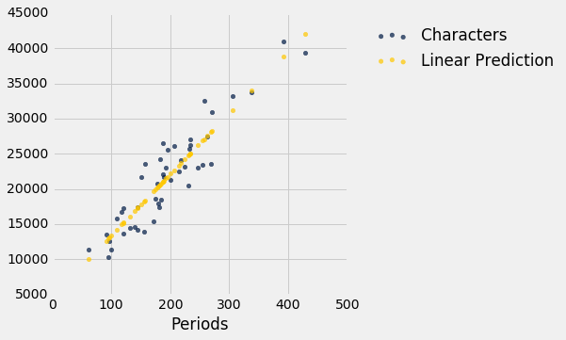``````actual = lw_with_predictions.column('Characters')
predicted = lw_with_predictions.column('Linear Prediction')
errors = actual - predicted
lw_with_predictions.with_column('Error', errors)
``````
Periods Characters Linear Prediction Error
189 21759 21183.6 575.403
188 22148 21096.6 1051.38
231 20558 24836.7 -4278.67
195 25526 21705.5 3820.54
255 23395 26924.1 -3529.13
140 14622 16921.7 -2299.68
131 14431 16138.9 -1707.88
214 22476 23358 -882.043
337 33767 34056.3 -289.317
185 18508 20835.7 -2327.69

（省略了 37 行）

``````lw_reg_slope = slope(little_women, 'Periods', 'Characters')
lw_reg_intercept = intercept(little_women, 'Periods', 'Characters')
print('Slope of Regression Line:    ', np.round(lw_reg_slope), 'characters per period')
print('Intercept of Regression Line:', np.round(lw_reg_intercept), 'characters')
lw_errors(lw_reg_slope, lw_reg_intercept)
Slope of Regression Line:     87.0 characters per period
Intercept of Regression Line: 4745.0 characters
``````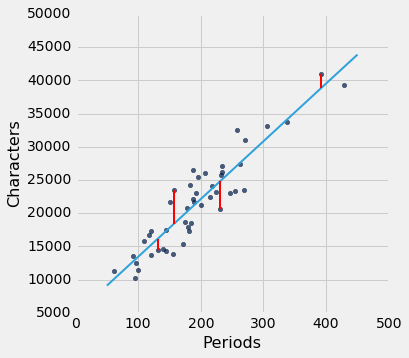``````lw_errors(50, 10000)
``````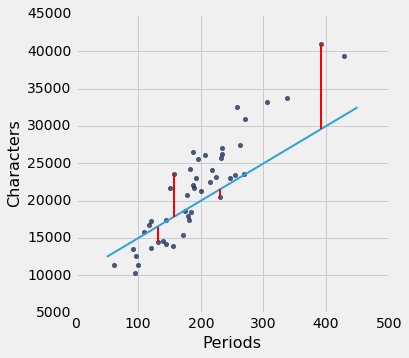``````lw_errors(-100, 50000)
``````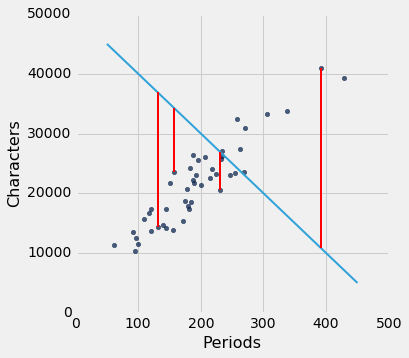### 使 RMSE 最小

• 要根据`x`估算`y`，可以使用任何你想要的直线。
• 每个直线都有估计的均方根误差。
• “更好”的直线有更小的误差。

``````def lw_rmse(slope, intercept):
lw_errors(slope, intercept)
x = little_women.column('Periods')
y = little_women.column('Characters')
fitted = slope * x + intercept
mse = np.mean((y - fitted) ** 2)
print("Root mean squared error:", mse ** 0.5)
lw_rmse(50, 10000)
Root mean squared error: 4322.16783177
````````````lw_rmse(-100, 50000)
Root mean squared error: 16710.1198374
````````````lw_rmse(90, 4000)
Root mean squared error: 2715.53910638
``````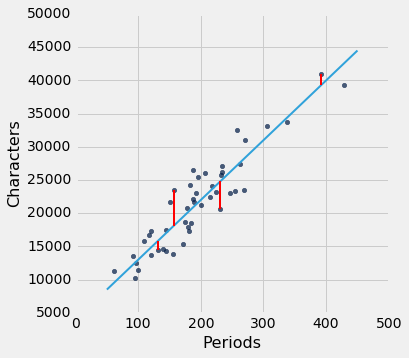``````lw_rmse(lw_reg_slope, lw_reg_intercept)
Root mean squared error: 2701.69078531
``````### 数值优化

``````def lw_mse(any_slope, any_intercept):
x = little_women.column('Periods')
y = little_women.column('Characters')
fitted = any_slope*x + any_intercept
return np.mean((y - fitted) ** 2)
``````

``````lw_mse(lw_reg_slope, lw_reg_intercept)**0.5
2701.690785311856
````````````lw_rmse(lw_reg_slope, lw_reg_intercept)
Root mean squared error: 2701.69078531
``````

``````lw_mse(-100, 50000)**0.5
16710.119837353752
``````

``````lw_mse(90, 4000)**0.5
2715.5391063834586
``````

`minimize`函数可用于寻找函数的参数，函数在这里返回其最小值。 Python 使用类似的试错法，遵循使输出值递减的变化量。

`minimize`的参数是一个函数，它本身接受数值参数并返回一个数值。 例如，函数`lw_mse`以数值斜率和截距作为参数，并返回相应的 MSE。

``````best = minimize(lw_mse)
best
array([   86.97784117,  4744.78484535])
``````

``````print("slope from formula:        ", lw_reg_slope)
print("slope from minimize:       ", best.item(0))
print("intercept from formula:    ", lw_reg_intercept)
print("intercept from minimize:   ", best.item(1))
slope from formula:         86.9778412583
slope from minimize:        86.97784116615884
intercept from formula:     4744.78479657
intercept from minimize:    4744.784845352655
``````

## 最小二乘回归

``````shotput = Table.read_table('shotput.csv')
shotput
``````
Weight Lifted Shot Put Distance
37.5 6.4
51.5 10.2
61.3 12.4
61.3 13
63.6 13.2
66.1 13
70 12.7
92.7 13.9
90.5 15.5
90.5 15.8

（省略了 18 行）

``````shotput.scatter('Weight Lifted')
``````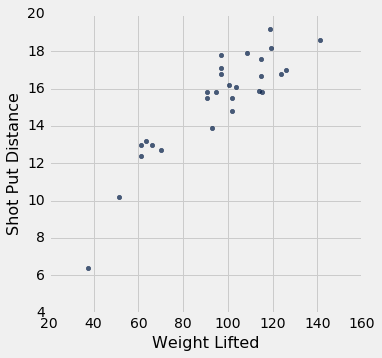``````slope(shotput, 'Weight Lifted', 'Shot Put Distance')
0.098343821597819972
intercept(shotput, 'Weight Lifted', 'Shot Put Distance')
5.9596290983739522
``````

``````def shotput_linear_mse(any_slope, any_intercept):
x = shotput.column('Weight Lifted')
y = shotput.column('Shot Put Distance')
fitted = any_slope*x + any_intercept
return np.mean((y - fitted) ** 2)
minimize(shotput_linear_mse)
array([ 0.09834382,  5.95962911])
````````````fitted = fit(shotput, 'Weight Lifted', 'Shot Put Distance')
shotput.with_column('Best Straight Line', fitted).scatter('Weight Lifted')
``````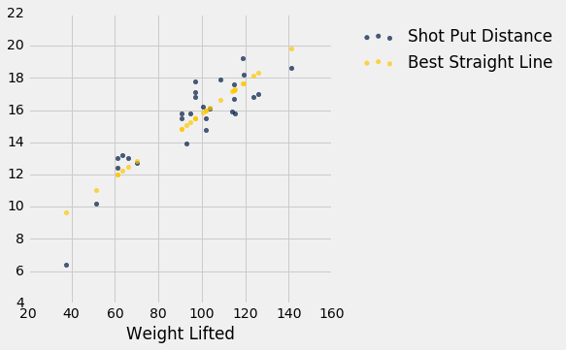### 非线性回归

``````f(x) = ax^2 + bx + c
``````

`a``b``c`是常数。

``````def shotput_quadratic_mse(a, b, c):
x = shotput.column('Weight Lifted')
y = shotput.column('Shot Put Distance')
fitted = a*(x**2) + b*x + c
return np.mean((y - fitted) ** 2)
``````

``````best = minimize(shotput_quadratic_mse)
best
array([ -1.04004838e-03,   2.82708045e-01,  -1.53182115e+00])
``````

``````(-0.00104)*(100**2) + 0.2827*100 - 1.5318
16.3382
``````

``````x = shotput.column(0)
shotput_fit = best.item(0)*(x**2) + best.item(1)*x + best.item(2)
``````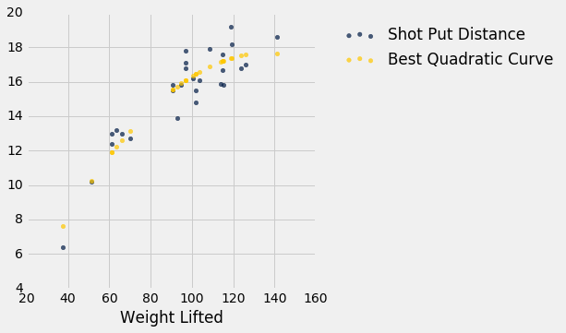## 视觉诊断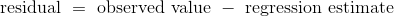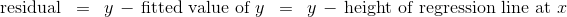`residual`函数计算残差。 该计算假设我们已经定义的所有相关函数：`standard_units``correlation``slope``intercept``fit`

``````def residual(table, x, y):
return table.column(y) - fit(table, x, y)
``````

``````heights = heights.with_columns(
'Fitted Value', fit(heights, 'MidParent', 'Child'),
'Residual', residual(heights, 'MidParent', 'Child')
)
heights
``````
MidParent Child Fitted Value Residual
75.43 73.2 70.7124 2.48763
75.43 69.2 70.7124 -1.51237
75.43 69 70.7124 -1.71237
75.43 69 70.7124 -1.71237
73.66 73.5 69.5842 3.91576
73.66 72.5 69.5842 2.91576
73.66 65.5 69.5842 -4.08424
73.66 65.5 69.5842 -4.08424
72.06 71 68.5645 2.43553
72.06 68 68.5645 -0.564467

（省略了 924 行）

``````def scatter_fit(table, x, y):
table.scatter(x, y, s=15)
plots.plot(table.column(x), fit(table, x, y), lw=4, color='gold')
plots.xlabel(x)
plots.ylabel(y)
scatter_fit(heights, 'MidParent', 'Child')
``````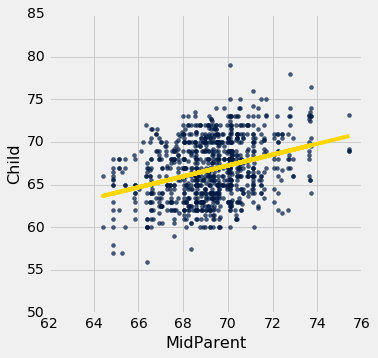``````def residual_plot(table, x, y):
x_array = table.column(x)
t = Table().with_columns(
x, x_array,
'residuals', residual(table, x, y)
)
t.scatter(x, 'residuals', color='r')
xlims = make_array(min(x_array), max(x_array))
plots.plot(xlims, make_array(0, 0), color='darkblue', lw=4)
plots.title('Residual Plot')
residual_plot(heights, 'MidParent', 'Child')
``````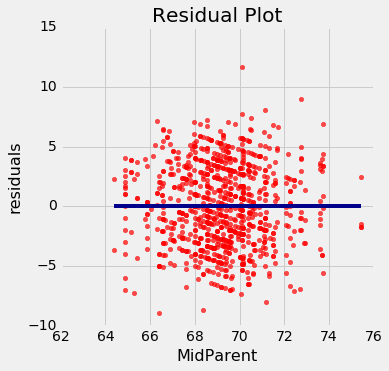### 回归诊断

``````def regression_diagnostic_plots(table, x, y):
scatter_fit(table, x, y)
residual_plot(table, x, y)
regression_diagnostic_plots(heights, 'MidParent', 'Child')
``````### 检测非线性``````dugong = Table.read_table('http://www.statsci.org/data/oz/dugongs.txt')
dugong = dugong.move_to_start('Length')
dugong
``````
Length Age
1.8 1
1.85 1.5
1.87 1.5
1.77 1.5
2.02 2.5
2.27 4
2.15 5
2.26 5
2.35 7
2.47 8

（省略了 17 行）

``````correlation(dugong, 'Length', 'Age')
0.82964745549057139
``````

``````regression_diagnostic_plots(dugong, 'Length', 'Age')
``````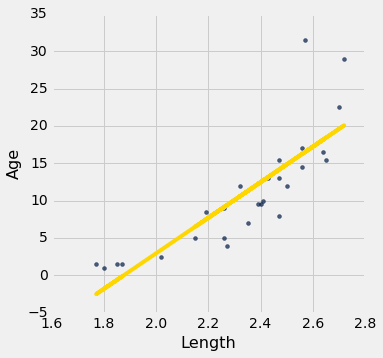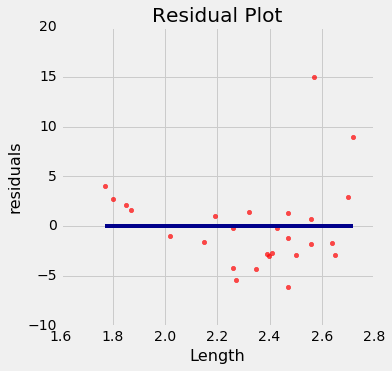### 检测异方差

``````regression_diagnostic_plots(hybrid, 'acceleration', 'mpg')
``````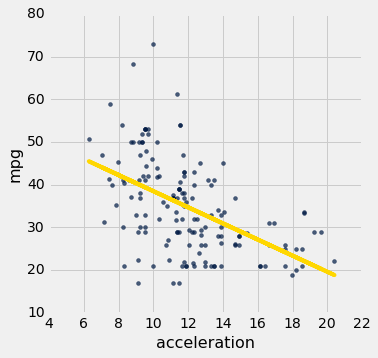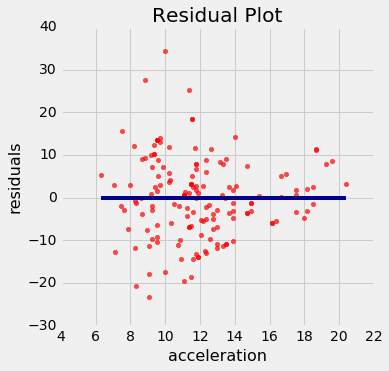## 数值诊断

### 残差图不展示形状

``````correlation(heights, 'MidParent', 'Residual')
-2.7196898076470642e-16
``````

``````round(correlation(heights, 'MidParent', 'Residual'), 10)
-0.0
dugong = dugong.with_columns(
'Fitted Value', fit(dugong, 'Length', 'Age'),
'Residual', residual(dugong, 'Length', 'Age')
)
round(correlation(dugong, 'Length', 'Residual'), 10)
0.0
``````

### 残差的均值

``````round(np.mean(heights.column('Residual')), 10)
0.0
``````

``````round(np.mean(dugong.column('Residual')), 10)
0.0
``````

### 残差的标准差``````np.std(heights.column('Residual'))
3.3880799163953426
``````

``````r = correlation(heights, 'MidParent', 'Child')
np.sqrt(1 - r**2) * np.std(heights.column('Child'))
3.3880799163953421
``````

``````r = correlation(hybrid, 'acceleration', 'mpg')
r
-0.5060703843771186
hybrid = hybrid.with_columns(
'fitted mpg', fit(hybrid, 'acceleration', 'mpg'),
'residual', residual(hybrid, 'acceleration', 'mpg')
)
np.std(hybrid.column('residual')), np.sqrt(1 - r**2)*np.std(hybrid.column('mpg'))
(9.4327368334302903, 9.4327368334302903)
``````

### 另一种解释`r`的方式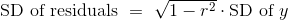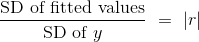``````scatter_fit(heights, 'MidParent', 'Child')
````````````correlation(heights, 'MidParent', 'Child')
0.32094989606395924
``````

``````np.std(heights.column('Fitted Value'))/np.std(heights.column('Child'))
0.32094989606395957
``````

``````correlation(hybrid, 'acceleration', 'mpg')
-0.5060703843771186
np.std(hybrid.column('fitted mpg'))/np.std(hybrid.column('mpg'))
0.5060703843771186
``````